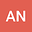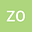2-Ruled hypersurfaces in Minkowski 4-space and their constructions via octonions
••Zehra Özdemir
Amasya University
Author Profile## Abstract

In this paper, we define three types of 2-Ruled hypersurfaces in the Minkowski 4-space $\mathbb{E}^4_1$. We obtain Gaussian and mean curvatures of the 2-ruled hypersurfaces of type-1 and type-2, and some characterizations about its minimality. We also deal with the first Laplace-Beltrami operators of these types of 2-Ruled hypersurfaces in $\mathbb{E}^4_1$. Moreover, the importance of this paper is that the definition of these surfaces by using the octonions in $\mathbb{E}^4_1$. Thus, this is a new idea and make the paper original. We give an example of 2-ruled hypersurface constructed by octonion and we visualize the projections of the images with MAPLE program. Furthermore, the optical fiber can be defined as a one-dimensional object embedded in the 4-dimensional Minkowski space $\mathbb{E}^4_1$. Thus, as a discussion, we investigate the geometric evolution of a linearly polarized light wave along an optical fiber by means of the 2-ruled hypersurfaces in a four-dimensional Minkowski space
29 May 2022Submitted to Mathematical Methods in the Applied Sciences
30 May 2022Assigned to Editor
30 May 2022Submission Checks Completed
08 Sep 2022Reviewer(s) Assigned